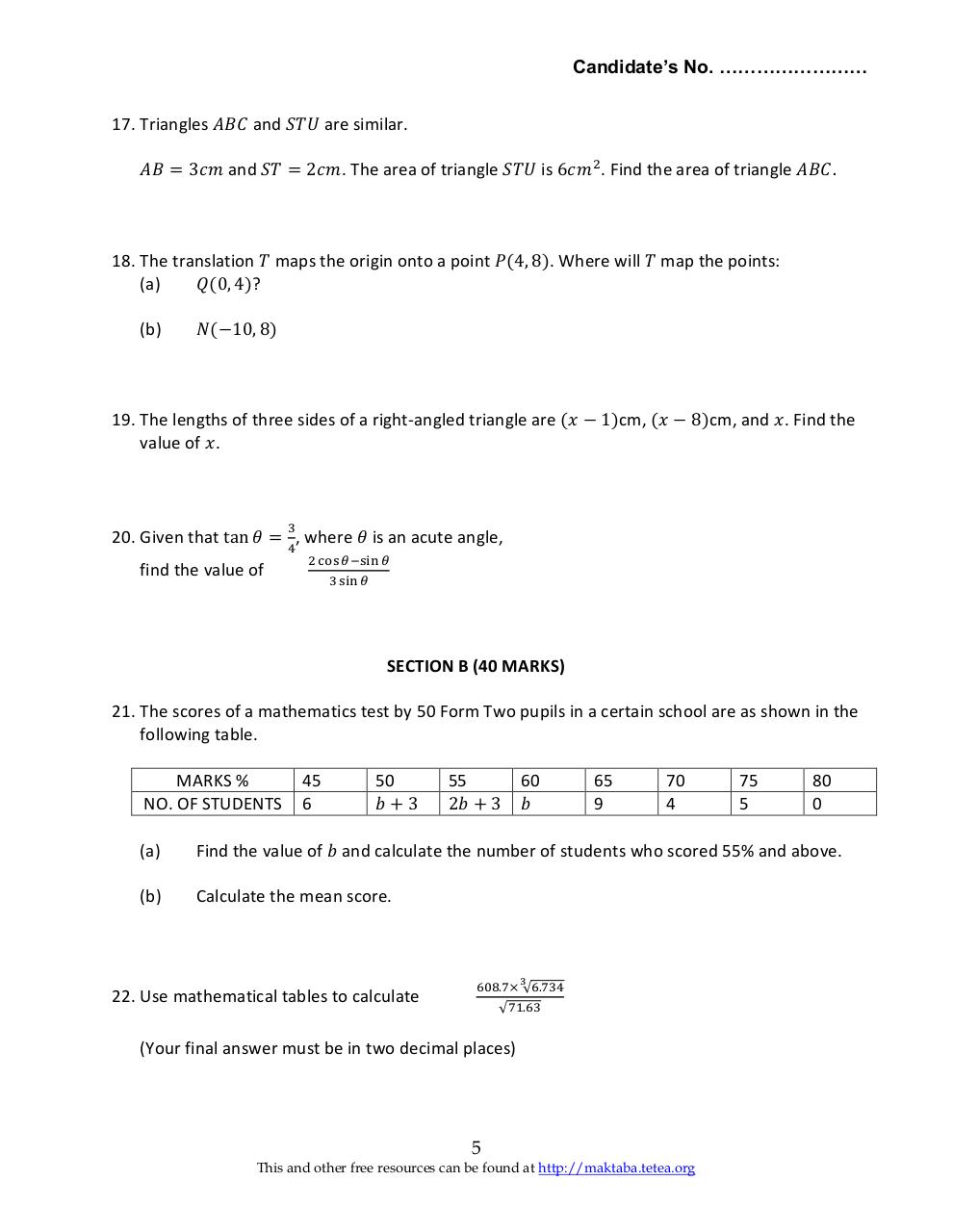# Basic Math 2005 F2.pdfPage 1 2 3 4 5 6

#### Text preview

Candidate’s No. ……………………
17. Triangles 𝐴𝐵𝐶 and 𝑆𝑇𝑈 are similar.
𝐴𝐵 = 3𝑐𝑚 and 𝑆𝑇 = 2𝑐𝑚. The area of triangle 𝑆𝑇𝑈 is 6𝑐𝑚2 . Find the area of triangle 𝐴𝐵𝐶.

18. The translation 𝑇 maps the origin onto a point 𝑃(4, 8). Where will 𝑇 map the points:
(a)
𝑄(0, 4)?
(b)

𝑁(−10, 8)

19. The lengths of three sides of a right-angled triangle are (𝑥 − 1)cm, (𝑥 − 8)cm, and 𝑥. Find the
value of 𝑥.

3

20. Given that tan 𝜃 = 4, where 𝜃 is an acute angle,
find the value of

2 cos 𝜃−sin 𝜃
3 sin 𝜃

SECTION B (40 MARKS)
21. The scores of a mathematics test by 50 Form Two pupils in a certain school are as shown in the
following table.
MARKS %
NO. OF STUDENTS

45
6

50
𝑏+3

55
2𝑏 + 3

60
𝑏

65
9

70
4

75
5

80
0

(a)

Find the value of 𝑏 and calculate the number of students who scored 55% and above.

(b)

Calculate the mean score.

3

22. Use mathematical tables to calculate

608.7× √6.734
√71.63